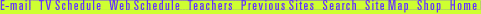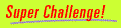## Using the Pythagorean Theorem

You've just been shown how the Pythagorean theorem works. Formal proofs show that the theorem is true not just for this triangle but for all right triangles. Once you know the equation a2 + b2 = c2 is true, then you can use it to solve all kinds of problems. Try the Pythagorean theorem with:Andrew Wiles | Math's Hidden Woman | Pythagorean Puzzle
Resources | Guide | Transcript | Proof Home